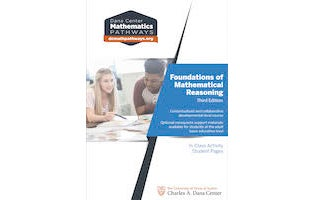Charles A Dana Center Web Catalog
Foundations of Mathematical Reasoning In-Class Activity Student Pages (third edition)

Foundations of Mathematical Reasoning In-Class Activity Student Pages (third edition)

Printed Book

The Dana Center's Foundations of Mathematical Reasoning (FMR) course is a semester-long developmental-level quantitative literacy-based course that surveys a variety of mathematical topics needed to prepare students for college-level statistics, quantitative reasoning, or algebra-intensive courses, as well as the workplace and as productive citizens.

The course is organized around big mathematical and statistical ideas, presented in contextualized, collaborative, in-class student activities. Concepts include numeracy (with an emphasis on estimation and fluency with large numbers); evaluating expressions and formulas; rates, ratios, and proportions; percentages; solving equations; linear models; data interpretations, including graphs and tables; verbal, algebraic, and graphical representations of functions; and exponential models.

The course helps students develop conceptual understanding, acquire multiple strategies for solving problems, and further develop the ability to read, write, and talk about mathematical concepts and applications.

Four contact hours per week, with the same instructor, are recommended.Item Price:
\$25.00

Item subject to sales tax.

Quantity: